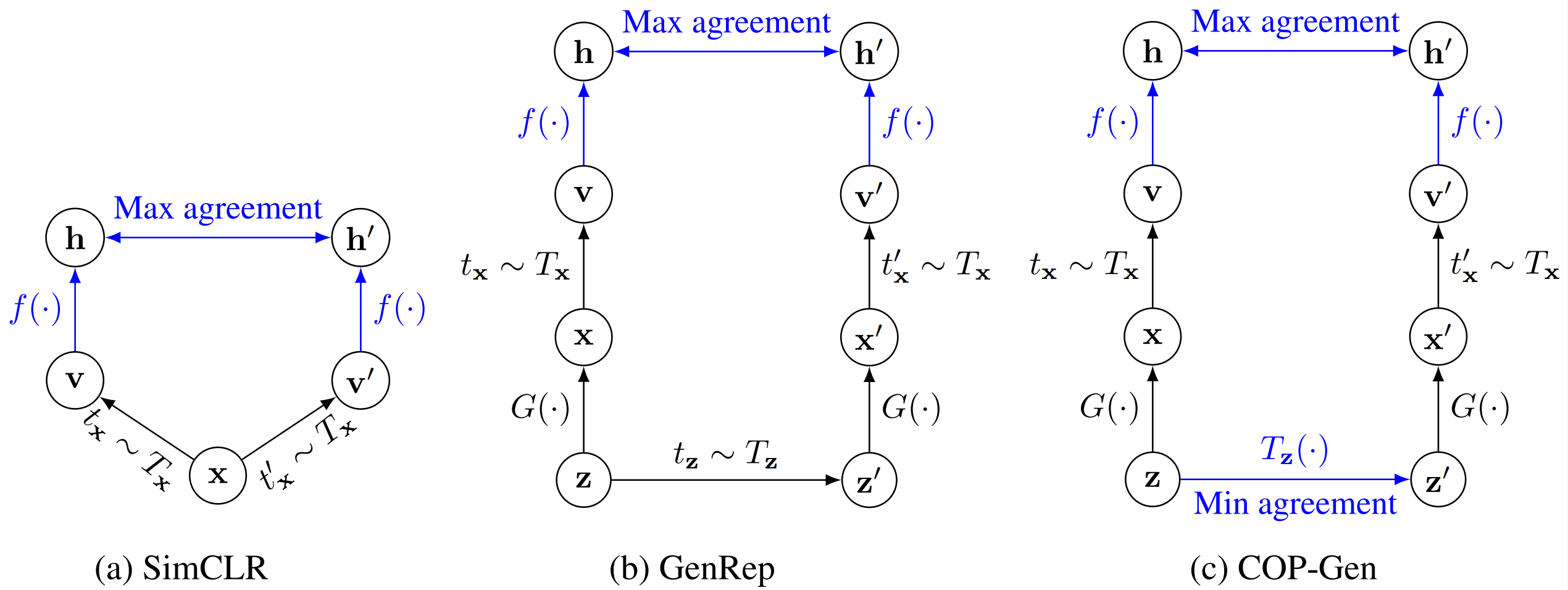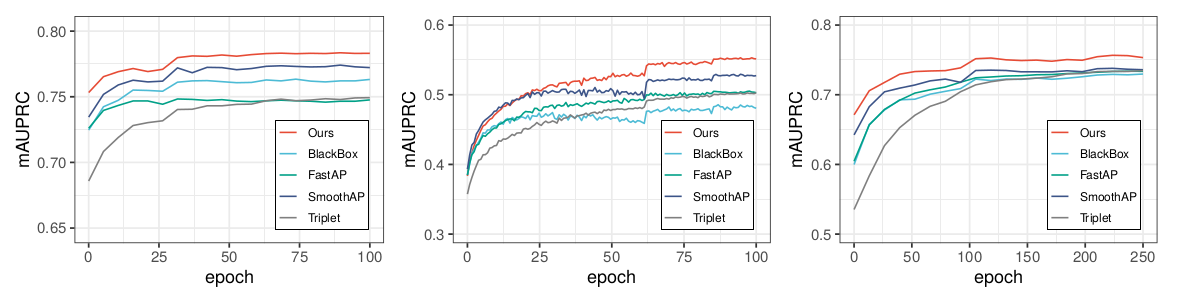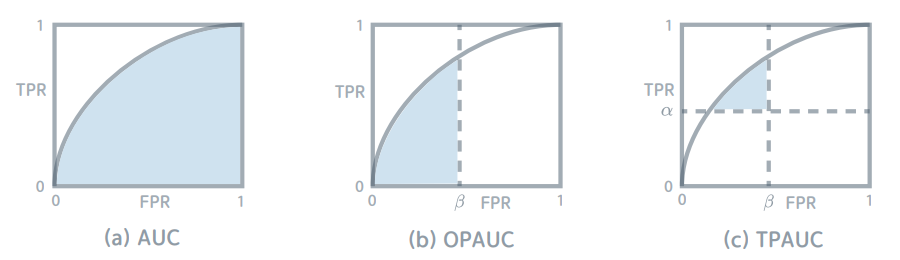Location：

# VIPL's 3 papers are accepted by NeurIPS 2022

Time: Sep 18, 2022

Congratulations! VIPL's 3 papers are accepted by NeurIPS 2022! The full name of NeurIPS 2022 is the Thirty-sixth Conference on Neural Information Processing Systems, which is one of the top conferences in artificial intelligence. NeurIPS 2022 will be held in New Orleans, USA from November 28 to December 9, 2022. The accepted papers are summarized as follows:

1. Optimal Positive Generation via Latent Transformation for Contrastive Learning (Yinqi Li, Hong Chang, Bingpeng Ma, Shiguang Shan, Xilin Chen)

Contrastive learning, which learns to contrast positive with negative pairs of samples, has been popular for self-supervised visual representation learning. Although great effort has been made to design proper positive pairs through data augmentation, few works attempt to generate optimal positives for each instance. Inspired by semantic consistency and computational advantage in latent space of pretrained generative models, this paper proposes to learn instance-specific latent transformations to generate Contrastive Optimal Positives (COP-Gen) for self-supervised contrastive learning. Specifically, we formulate COP-Gen as an instance-specific latent space navigator which minimizes the mutual information between the generated positive pair subject to the semantic consistency constraint. Theoretically, the learned latent transformation creates optimal positives for contrastive learning, which removes as much nuisance information as possible while preserving the semantics. Empirically, using generated positives by COP-Gen consistently outperforms other latent transformation methods and even real-image-based methods in self-supervised contrastive learning.Figure 1 Comparison of positive pairs generalization methods

2. Exploring the Algorithm-Dependent Generalization of AUPRC Optimization with List Stability (Peisong Wen, Qianqian Xu, Zhiyong Yang, Yuan He, Qingming Huang)

Area Under the Precision-Recall Curve (AUPRC) is a widely used metric in the machine learning community, especially in long-tailed classification and learning to rank. Although AUPRC optimization has been applied in various scenarios, the algorithm-dependent generalization performance is still blank. To fill this gap, in this paper we aim to design a stochastic optimization framework for AUPRC with a provable algorithm-dependent generalization. The target is challenging in three aspects: (a) Most AUPRC stochastic estimators are biased with a biased sampling rate; (b) AUPRC involves a non-decomposable listwise loss, making the stability-based framework to analyze the generalization infeasible; (c) AUPRC optimization is a compositional optimization problem, which is typically solved by alternateupdates. This brings more complicated stability calculations. In search of a solution to (a), we propose a sampling-rate-invariant asymptotically unbiased stochastic estimator based on a reformulation of AUPRC. Facing challenge (b), we extend instancewise model stability to listwise model stability, and correspondingly put forward the generalization via the stability of listwise problems. As for challenge (c), we consider state transition matrices of these variables, and simplify the calculations of the stability with matrix spectrum. Empirical studies on three image retrieval datasets validate the effectiveness and soundness of the proposed framework.Figure 2 Convergence results on test sets of three image retrieval datasets

3. Asymptotically Unbiased Instance-wise Regularized Partial AUC Optimization: Theory and Algorithm (Huiyang Shao, Qianqian Xu, Zhiyong Yang, Shilong Bao, Qingming Huang)

Compared with traditional imbalanced data classification problems, application scenarios such as medical diagnosis, spam filtering, and risky financial account detection tend to train models to obtain a higher True Positive Rate (TPR) within a lower False Positive Rate (FPR) interval, in other words, these application scenarios focus more on learning hard samples, while the AUC (Area Under the ROC Curve) metrics focus more on the overall performance, which is not consistent with the existing requirements. Therefore, in this paper, we consider optimizing the one-way partial AUC within the lower false positive rate interval, called OPAUC, and the two-way partial AUC within the lower false positive rate and higher true positive rate interval, called TPAUC. The following difficulties exist in optimizing the partial AUC: (a) there is a quantile function in the objective function and the function is not differentiable, so it is not possible to optimize the partial AUC in an end-to-end manner directly; (b) the existing optimization of partial AUC metrics uses a pair-wise loss function, which is not suitable for an end-to-end approach. To solve the above problems, this paper proposes an instance-wise minimax deep learning framework (Asymptotically Unbiased Instance-wise Regularized Partial AUC Optimization) to optimize the partial AUC. First, to overcome difficulty (a), we use a differentiable surrogate loss, called ATK loss (Average Top-k Loss), which obtains the same result as the original quantile function at the optimal solution. To address difficulty (b), we use an existing instance-wise AUC optimization formulation. With the ATK loss, we extend this formulation to partial AUC optimization. Experiments on nine benchmark datasets show that the proposed method in this paper can effectively improve the partial AUC.Figure 3 The partial AUC optimized in this paper